# RS Aggarwal Class 8 Solutions Chapter 21 Data Handling Ex 21B

## RS Aggarwal Class 8 Solutions Chapter 21 Data Handling Ex 21B

These Solutions are part of RS Aggarwal Solutions Class 8. Here we have given RS Aggarwal Solutions Class 8 Chapter 21 Data Handling Ex 21B.

Other Exercises

Question 1.
Solution:
Frequency distribution table is given below: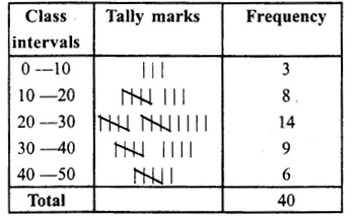Question 2.
Solution:
Arranging the given data in increasing order:
312, 324, 356, 365, 378, 400, 435, 472, 506, 548, 565, 570, 584, 596, 617, 630, 674, 685, 700, 736, 745, 754, 763, 776, 780.
Now frequency distribution table is given below :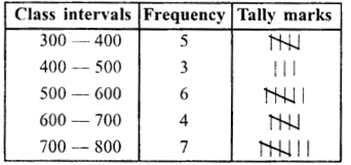Question 3.
Solution:
Frequency Distribution table is given below: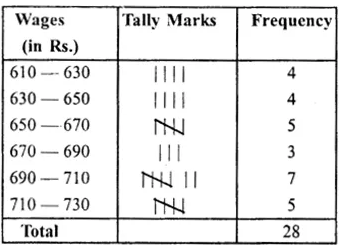Question 4.
Solution:
Frequency distribution table is given below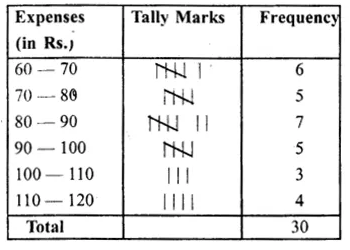Question 5.
Solution:
Frequency table is given below :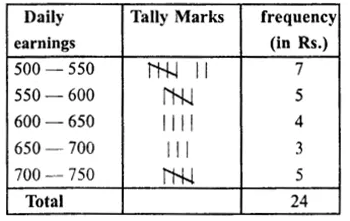Question 6.
Solution: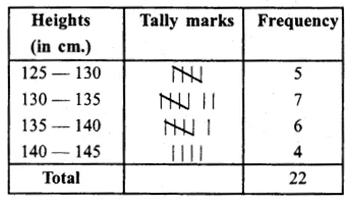Hope given RS Aggarwal Solutions Class 8 Chapter 21 Data Handling Ex 21B are helpful to complete your math homework.

If you have any doubts, please comment below. Learn Insta try to provide online math tutoring for you.